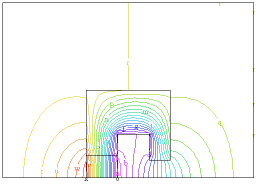﻿ Sample Problems > Applications > Magnetism > permanent_magnet

# permanent_magnet

Navigation:  Sample Problems > Applications > Magnetism >

# permanent_magnet{ PERMANENT_MAGNET.PDE

This example demonstrates the implementation of permanant magnets in magnetic field problems.

FlexPDE integrates second-order derivative terms by parts, which creates surface integral

terms at cell boundaries.

By including magnetization vectors inside the definition of H, these surface terms correctly

model the effect of magnetization through jump terms at boundaries.

If the magnetization terms are listed separately from H, they will be seen as piecewise

constant in space, and their derivatives will be deleted.

See the Electromagnetic Applications section for further discussion.

}

 Title 'A PERMANENT-MAGNET PROBLEM'   Variables    A { z-component of Vector Magnetic Potential }   Definitions    mu = 1    S = 0             { current density }    Px = 0             { Magnetization components }    Py = 0    P = vector(Px,Py) { Magnetization vector }    H = (curl(A)-P)/mu { Magnetic field }      y0 = 8             { Size parameter }   Materials 'Magnet' : Py = 10 'Other'  : mu = 5000   Initial values     A = 0   Equations         A : curl(H) + S = 0   Boundaries   Region 1     start(-40,0)     natural(A) = 0 line to (80,0)value(A) = 0   line to (80,80) to (-40,80) to close

Region 2

use material 'Other'

start(0,0)

line to (15,0) to (15,20) to (30,20) to (30,y0) to (40,y0) to (40,40)

to (0,40) to close

Region 3   { the permanent magnet }

use material 'Magnet'

start (0,0) line to (15,0) to (15,10) to (0,10) to close

Monitors

contour(A)

Plots

grid(x,y)

vector(dy(A),-dx(A)) as 'FLUX DENSITY B'

vector((dy(A)-Px)/mu, (-dx(A)-Py)/mu) as 'MAGNETIC FIELD H'

contour(A) as 'Az MAGNETIC POTENTIAL'

surface(A) as 'Az MAGNETIC POTENTIAL'

End